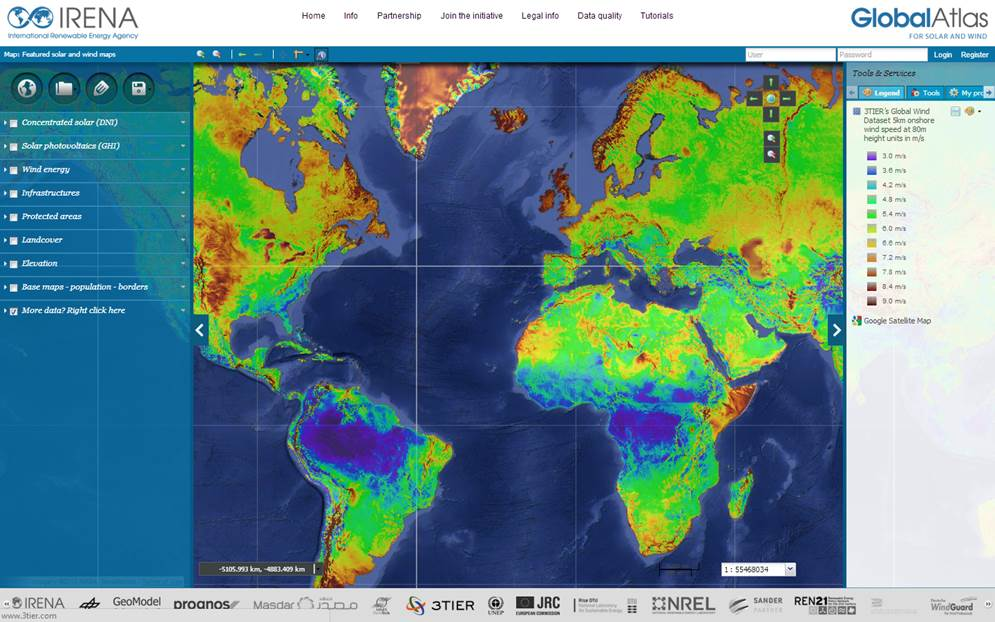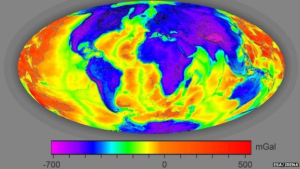#Eye on the Energy Sources of the Future

Geothermal energy prospectors have long used gravity meters in their search for the right subsurface characteristics.  But these have been point measurements.  GOCE now provides this information across the World at a resolution never before achieved on that scale.

Information about the variations in gravity across Earth might help prospectors find promising locations where sub-surface heat can be exploited to generate electricity in the future.

Gravity surveys are used for modelling the Earth’s crustal structure, for locating bedrock fractures, buried topographic valleys, subsurface voids such as abandoned mines, caverns, and sinkholes, and for determining overburden thickness and depth to bedrock.

The International Renewable Energy Agency (IRENA) released the information in a new online Global Atlas for Renewable Energy.

## Gravity Anomalies, Mineshaft Maps and Gals…

A gravity anomaly is the difference between the observed acceleration of a planet’s gravity and a value predicted from a model. A location with a positive anomaly exhibits more gravity than predicted, while a negative anomaly exhibits a lower value than predicted.The anomaly is the body or effect that causes the deviation from the “ideal” gravity model. Many data corrections must be made to the measured gravity value in order to extract the response of the local anomaly, or local geology, which is typically the goal of applied geophysics.

Gravity (gravitational acceleration) decreases as an inverse square law with the distance at which the measurement is made from the mass.$\boldsymbol{g}=\frac {Gm}{r^{2}}$

Lateral variations in gravity anomalies are related to anomalous density distributions within the Earth.  Gravity measures help us to understand the internal structure of the planet.Gravity anomaly map of an abandoned mine.

The gravity method involves measuring the acceleration due to the Earth’s gravitational field.

A gravity meter or gravimeter is used to measure the variations in the Earth’s gravitational field. The variations in gravity are due to lateral changes in the density of the subsurface rocks in the vicinity of the measuring point.A gravity profile from above map and its interpreted model. Source: New Jersey Geological and Water Survey.

Because the density variations are very small and uniform, the gravimeters are very sensitive and they measure one part in 100 million of the Earth’s gravity field (980 gals or 980,000 milligals) in units of milligals or microgals.

At the Earth’s equator,$\frac {2 \boldsymbol{g}}{r}=0.3086 \mathrm{mGal m^{-1}}$.

The gal, sometimes called galileo, (symbol Gal) is a unit of acceleration used extensively in the science of Gravimetry.  The gal is defined as 1 centimetre per second squared (1 cm/s2).  The milligal (mGal) and microgal (µGal) refer to one thousandth and one millionth of a gal, respectively.

# Free Air and Bouguer

For IRENA, GOCE researchers provided two gravity models: “Free Air” and “Bouguer”.

The Free Air gravity map provides insights into particular structures that might favour geothermal energy.  The Bouguer map gives details of crustal thickness.

In Geophysics, the free air gravity anomaly, or free-air anomaly, is the measured gravity anomaly after a free-air correction is applied to correct for the elevation at which a measurement is made.  The free-air correction does so by adjusting these measurements of gravity to what would have been measured at a point of reference.

For Earth, this reference level is commonly taken as the mean sea level.

## Free-Air Anomaly Correction

The free air correction is calculated from Newton’s Law, as a rate of change of gravity with distance.

Since gravity is$\boldsymbol{g}=\frac {Gm}{r^{2}}$.

The rate of change of gravity is$\frac{d \boldsymbol g}{dr} = \frac {-2Gm}{r^{3}}=\frac{-2g}{r}$.

So, the free air correction is given by:$\delta{g_F}=\frac {2g}{r} \times h$.

The free-air correction is the amount that must be added to a measurement taken at height h to correct it to the reference level point.

## Bouguer Anomaly CorrectionThe Bouguer anomaly map provides information on the Earth’s crust thickness. Source: ESA/IRENA

The Bouguer anomalies usually are negative in the mountains because of isostasy – the rock density of their roots is lower, compared with the surrounding Earth’s mantle.

The Bouguer anomaly is corrected for the height at which it is measured and the attraction of terrain.  It is related to the observed gravity gobs as follows:$g_B = g_{obs} - g_{\lambda} + \delta g_F - \delta g_B$$g_B = g_F - \delta g_B$

Here, gB is the Bouguer anomaly, gF is the free-air gravity anomaly, gλ is the correction for latitude (because the Earth is not a perfect sphere) and δ gF is the free-air correction and δ gB is the correction for terrain called the Bouguer reduction.

The gravitational acceleration g outside a Bouguer plate is perpendicular to the plate and points towards it, with a magnitude 2πG times the mass per unit area, where G is the gravitational constant, and independent of the distance to the plate (Gauss’s Law for Gravity and Newton’s Law of Gravity).

G = 6.67 × 10−11 N m² kg−2, so g = 4.191 × 10−10 N m² kg−2  x [mass per unit area].

Using 1 Gal = 0.01 m/s², we get 4.191 × 10−5 mGal m² kg−1 x [mass per unit area].

For a mean rock density (~2.67 g/cm³), this gives 0.1119 mGal/m.

Assuming that the terrain is approximated by an infinite flat plane, the Bouguer reduction for a Bouguer plate of thickness H is$\delta \boldsymbol{g_B} = 2 \pi \rho \boldsymbol G H$,

where ρ is the density of the material and G is the constant of gravitation.

#### On Earth, the effect on gravity of elevation is a 0.3086 mGal/m decrease when going up, minus the gravity of the Bouguer plate, giving the Bouguer gradient of 0.1967 mGal/m.

For a mass distribution with the density depending on one Cartesian coordinate z only, gravity for any z is 2πG times the difference in mass per unit area on either side of this z value.  A combination of two equal parallel infinite plates does not produce any gravity inside.

Typical anomalies in the Central Alps are −150 milligals (−1.5 mm/s²).  Rather local anomalies are used in applied geophysics: if they are positive, this may indicate metallic ores.  At scales between entire mountain ranges and ore bodies, Bouguer anomalies may indicate rock types.

For example, the northeast-southwest trending high across central New Jersey represents a graben of Triassic age largely filled with dense basalts.  Salt domes are typically expressed in gravity maps as lows.  Salt has a low density compared to the rocks the dome intrudes.  Anomalies can help distinguish sedimentary basins whose fill differs in density from that of the surrounding region.

# Geothermal TechnologyNatural Geothermic Reservoir Diagram      Source: energy.gov

Clean, renewable, and popular, geothermal energy can be harnessed from almost anywhere in the World to produce heat and electricity.  Historically recognised as hot springs, geothermal energy is now commonly used today for residential heating and cooling, and electricity generation.  Although geothermal is not the most widely used source of energy, it is by far one of the most efficient and sustainable systems today.

Prospectors still need further information to find the heat reservoirs – but the new online portal is a good place to start, particularly for countries where local geological surveys may be very difficult to conduct and are therefore incomplete.The Bjarnarflag Geothermal Power Plant is Iceland’s first.  The plant was built in 1969. It is located in North Iceland, within the historic Lake Myvatn area.

Free Air helps scientists understand the structures, because to have an exchange of high temperatures at low levels and the surface, you want to use existing fractures.  These fractures are found at contacts between different rock types.

The Free Air model maps these contacts.

Bouguer tells them where there are crustal thickness variations, and where the mantle is coming up to the surface.  For geothermal energy exploitation, you want a thin crust because this is where the temperature gradients will be the highest.

# GOCE does it again!

The data comes from Europe’s GOCE satellite, which mapped Earth’s gravity field from 2009 to 2013 at high resolution.  The prime mission goal of GOCE was to acquire gravity field information to investigate how ocean currents move, but it has proven to have many additional solid Earth applications too.Towards the end of the satellite’s life in November 2013, the altitude of the spacecraft was lowered to improve the resolution.  This particular geothermal energy application demonstrates how it was really worth doing.

GOCE’s keen sensing and the observations it made of Earth from space, are now expected to help pinpoint the search for prime spots to put future geothermal energy power stations.

With GOCE, we are able to see the gravity anomalies at a resolution of between 70 and 80km!